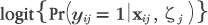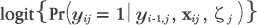# Errata for Multilevel and Longitudinal Modeling Using Stata, Second Edition

The errata for Multilevel and Longitudinal Modeling Using Stata, Second Edition, are provided below. Click here for an explanation of how to read an erratum. Click here to learn how to determine the printing number of a book.

 (1,2) Chapter 1, p. 17, third line under equation
 Here t = 99.53, ... Here t = 10.09, ...
 (1) Chapter 3, p. 132, last line of third paragraph
 SES) is often called a contextual effect or compositional effect. SES) is often called a contextual effect in contrast to compositional effect.
 (1,2) Chapter 4, p. 165, first two histogram commands at the bottom of the page
 . histogram reff1 if pickone==1, normal xtitle(Predicted random slopes) . histogram reff2 if pickone==1, normal xtitle(Predicted random intercepts) . histogram ebs if pickone==1, normal xtitle(Predicted random slopes) . histogram ebi if pickone==1, normal xtitle(Predicted random intercepts)
 (1,2) Chapter 4, p. 167, last group of Stata examples on page
 . gsort +reffm1 -f . generate rank = sum(f) . generate labpos = reffm1 + 1.96*reffs1 + .4 . serrbar reffm1 reffs1 rank if f==1, addplot(scatter labpos rank, mlabel(school) msymbol(none) mlabpos(0)) scale(1.96) xtitle(Rank) ytitle(Prediction) legend(off) . gsort +reffm1 -pickone . generate rank = sum(pickone) . generate labpos = reffm1 + 1.96*reffs1 + .4 . serrbar reffm1 reffs1 rank if pickone==1, addplot(scatter labpos rank, mlabel(school) msymbol(none) mlabpos(0)) scale(1.96) xtitle(Rank) ytitle(Prediction) legend(off)
 (1) Chapter 5, p. 196, three lines above section 5.5.5
 can be eliminated by double differencing or “difference-in-difference”, .... can be eliminated by double differencing, ....
 (1) Chapter 6, p. 281, exercise 6.7.2, left-hand-side of the equation(1) Chapter 6, p. 284, exercise 6.9.6c
 c. Run the gllamm command from step 3 ... c. Run the gllamm command from step 2 ...
 (1) Chapter 6, p. 284, exercise 6.9.6d
 d. Compare the estimates with those from step 3 ... d. Compare the estimates with those from step 2 ...
 (1) Chapter 10, p. 467, exercise 10.4.2
 2. Obtain the estimated intraclass correlations of the latent responses for ... 2. Obtain the estimated intraclass correlations for ...
 (1) Chapter 10, p. 470, exercise 10.9.5
 5. Perform likelihood-ratio tests for the two variance components using a 5% level of significance. 5. Perform likelihood-ratio tests for each of the variance components using a 5% level of significance.
 (1) Chapter 11, p. 478, first sentence of paragraph above section 11.3.4
 The reason for choosing year to be at level 2 and to have a random intercept for each firm at level 3 is to minimize the computational burden. The reason for choosing year to be at level 3 and to have a random intercept for each firm at level 2 is to minimize the computational burden.
 (1) Chapter 11, p. 502, exercise 11.2.6
 6. Fit the model ... Explain how and why the estimated coefficient of lnfuel changes compared with the model that treats year as random. 6. Fit the model ... Explain how and why the estimated coefficient of log fuel price changes compared with the model that treats year as random.